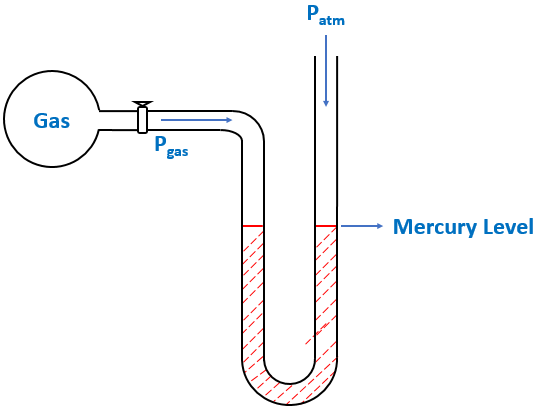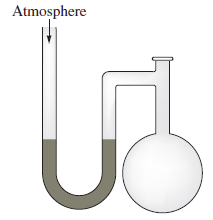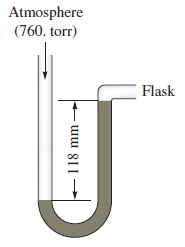# Problem: A diagram for an open-tube manometer is shown below.If the flask is open to the atmosphere, the mercury levels are equal. For each of the following situations where a gas is contained in the flask, calculate the pressure in the flask in torr, atmospheres, and pascals.a.

###### FREE Expert Solution

We’re being asked to determine the pressure of a gas (in atm, torr, and Pa) using an open-end manometer.

A manometer allows you to determine the pressure of a gas (Pgas) sample by using the atmospheric pressure (Patm) and the difference in the height of mercury (h).

Different conditions for an open-end manometer are illustrated below:

When Pgas is pushing the same force as Patm$\overline{){{\mathbf{P}}}_{\mathbf{g}\mathbf{a}\mathbf{s}}{\mathbf{=}}{{\mathbf{P}}}_{\mathbf{a}\mathbf{t}\mathbf{m}}}$

When Pgas is pushing harder than Patm

99% (239 ratings)###### Problem Details

A diagram for an open-tube manometer is shown below.If the flask is open to the atmosphere, the mercury levels are equal. For each of the following situations where a gas is contained in the flask, calculate the pressure in the flask in torr, atmospheres, and pascals.

a.